Home | | Resource Management Techniques | Algebraic Sensitivity Analysis-Changes in the Right-Hand Side

# Algebraic Sensitivity Analysis-Changes in the Right-Hand Side

In Previous Section , we used the graphical solution to determine the dual prices (the unit worths of resources) and their feasibility ranges.

Algebraic Sensitivity Analysis-Changes in the Right-Hand Side

In Previous Section , we used the graphical solution to determine the dual prices (the unit worths of resources) and their feasibility ranges. This section extends the analysis to the general LP model. A numeric example (the TOYCO model) will be used to facilitate the presentation.

Example 3.6-2   (TOYCO Model)

TOYCO assembles three types of toys-trains, trucks, and cars-using three operations. The daily limits on the available times for the three operations are 430,460, and 420 minutes, respectively, and the revenues per unit of toy train, truck, and car are \$3, \$2, and \$5, respectively. The as-sembly times per train at the three operations are 1, 3, and 1 minutes, respectively. The corresponding times per train and per car are (2,0,4) and (1,2,0) minutes (a zero time indicates that the operation is not used).

Letting x1, x2, and x3 represent the daily number of units assembled of trains, trucks, and cars, respectively, the associated LP model is given as:The solution recommends manufacturing 100 trucks and 230 cars but no trains. The associated revenue is \$1350.

Determination of Dual Prices. The constraints of the model after adding the slack variables x4, x5, and x6 can be written as follows: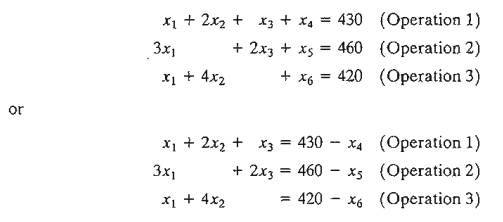With this representation, the slack variables have the same units (minutes) as the operation times. Thus, we can say that a one-minute decrease in the slack variable is equivalent to a one-minute increase in the operation time.

We can use the information above to determine the dual prices from the z-equa-tion in the optimal tableau:Given that a decrease in the value of a slack variable is equivalent to an increase in its operation time, we getThis equation reveals that (1) a one-minute increase in operation 1 time increases z by \$1, (2) a one-minute increase in operation 2 time increases z by \$2, and (3) a one-minute increase in operation 3 time does not change z.

To summarize, the z-row in the optimal tableau:The zero dual price for operation 3 means that there is no economic advantage in allocating more production time to this operation. The result makes sense because the resource is already abundant, as is evident by the fact that the slack variable associated with Operation 3 is positive (= 20) in the optimum solution. As for each of Operations 1 and 2, a one minute increase will improve revenue by \$1 and \$2, respectively. The dual prices also indicate that, when allocating additional resources, Operation 2 may be given higher priority because its dual price is twice as much as that of Operation 1.

The computations above show how the dual prices are determined from the optimal tableau for constraints. For constraints, the same idea remains applicable except that the dual price will assume the opposite sign of that associated with the constraint. As for the case where the constraint is an equation, the determination of the dual price from the optimal simplex tableau requires somewhat "involved" calcula-tions as will be shown in Chapter 4.

Determination of the Feasibility Ranges. Having determined the dual prices, we show next how the feasibility ranges in which they remain valid are determined. Let DI , Dz, and D3 be the changes (positive or negative) in the daily manufacturing time allocated to operations 1,2, and 3, respectively. The model can be written as follows:We will consider the general case of making the changes simultaneously. The special cases of making change one at a time are derived from these results.

The procedure is based on recomputing the optimum simplex tableau with the modified right-hand side and then deriving the conditions that will keep the solution feasible-that is, the right-hand side of the optimum tableau remains nonnegative. To show how the right-hand side is recomputed, we start by modifying the Solution column of the starting tableau using the new right-hand sides: 430 + D1 460 + D2, and 420 + D3 . The starting tableau will thus appear as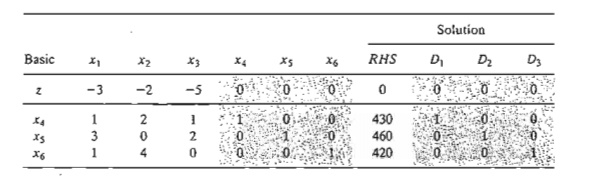The columns under D1 D2 and D3 are identical to those under the starting basic columns x4,x5, and x6. This means that when we carry out the same simplex iterations as in the original model, the columns in the two groups must come out identical as well. Effectively, the new optimal tableau will becomeThe new optimum tableau provides the following optimal solution:Interestingly, as shown earlier, the new z-value confirms that the dual prices for operations 1,2, and 3 are 1,2, and 0, respectively.

The current solution remains feasible so long as all the variables are nonnegative, which leads to the following feasibility conditions:Any simultaneous changes D1, D2, and D3 that satisfy these inequalities will keep the solution feasible. If all the conditions are satisfied, then the new optimum solution can be found through direct substitution of D1,D2  and D3 in the equations given above.

To illustrate the use of these conditions, suppose that the manufacturing time available for operations 1,2, and 3 are 480,440, and 410 minutes respectively. Then, Dl = 480 - 430 = 50, D2 = 440 - 460 = -20, and D3 = 410 - 420 = -10. Substituting in the feasibility conditions, we get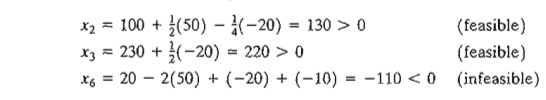The calculations show that x6 < 0, hence the current solution does not remain feasible. Additional calculations will be needed to find the new solution. These calculations are discussed in Chapter 4 as part of the post-optimal analysis.

Alternatively, if the changes in the resources are such that D1 = -30, D2 = -12, and D3 = 10, then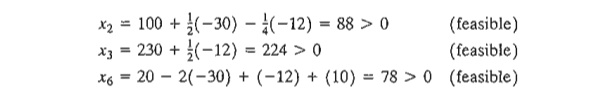The new feasible solution is x1 = 88, x3 = 224, and x6 = 68 with z = 3(0) + 2(88) + 5(224) = \$1296. Notice that the optimum objective value can also be computed as z = 1350 + 1( -30) + 2( -12) = \$1296.

The given conditions can be specialized to produce the individual feasibility ranges that result from changing the resources one at a time (as defined in Section 3.6.1).

Case 1. Change in operation 1 time from 460 to 460 + D1 minutes. This change is equiv-alen to setting D2 = D3 = 0 in the simultaneous conditions, which yields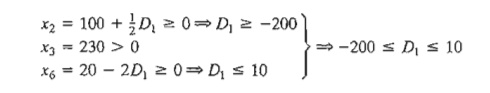Case 2. Change in operation 2 time from 430 to 430 + D2 minutes. This change is equivalent to setting D1 = D3 = 0 in the simultaneous conditions, which yieldsCase 3. Change in operation 3 time from 420 to 420 + D3 minutes. This change is equivalent to setting D1 = D2 = 0 in the simultaneous conditions, which yieldsWe can now summarize the dual prices and their feasibility ranges for the TOYCO model as follows:It is important to notice that the dual prices will remain applicable for any simultaneous changes that keep the solution feasible, even if the changes violate the indi-vidual ranges. For example, the changes D1 = 30, D2 = -12, and D3 = 100, will keep the solution feasible even though D1 = 30 violates the feasibility range -200 ≤ Dl ≤ 10, as the following computations show:This means that the dual prices will remain applicable, and we can compute the new optimum objective value from the dual prices as z = 1350 + 1(30) + 2( -12) + 0(100) = \$1356

The results above can be summarized as follows:

1. The dual prices remain valid so long as the changes Di , i = 1,2, ... , m, in the right-hand sides of the constraints satisfy all the feasibility conditions when the changes are simultaneous or fall within the feasibility ranges when the changes are made individually.

2. For other situations where the dual prices are not valid because the simultaneous feasibility conditions are not satisfied or because the individual feasibility ranges are violated, the recourse is to either re-solve the problem with the new values of Di or apply the post-optimal analysis presented in Chapter 4.

PROBLEM SET 3.6et

1. In the TOYCO model, suppose that the changes DI , Dz, and D3 are made simultaneously in the three operations.

(a) If the availabilities of operations 1,2, and 3 are changed to 438,500, and 410 minutes, respectively, use the simultaneous conditions to show that the current basic solution remains feasible, and determine the change in the optimal revenue by using the optimal dual prices.

(b) If the availabilities of the three operations are changed to 460,440, and 380 minutes, respectively, use the simultaneous conditions to show that the current basic solution becomes infeasible.

*2.     Consider the TOYCO model.

(a)     Suppose that any additional time for operation 1 beyond its current capacity of 430 minutes per day must be done on an overtime basis at \$50 an hour. The hourly cost includes both labor and the operation of the machine. Is it economically advantageous to use overtime with operation I?

(b)     Suppose that the operator of operation 2 has agreed to work 2 hours of overtime daily at \$45 an hour. Additionally, the cost of the operation itself is \$10 an hour. What is the net effect of this activity on the daily revenue?

(c)      Is overtime needed for operation 3?

(d) Suppose that the daily availability of operation 1 is increased to 440 minutes. Any overtime used beyond the current maximum capacity will cost \$40 an hour. Deter-mine the new optimum solution, including the associated net revenue.

(e) Suppose that the availability of operation 2 is decreased by 15 minutes a day and that the hourly cost of the operation during regular time is \$30. Is it advantageous to decrease the availability of operation 2?

3. A company produces three products, A, B, and C. The sales volume for A is at least 50% of the total sales of all three products. However, the company cannot sell more than 75 units of A per day. The three products use one raw material, of which the maxi-mum daily availability is 240 lb. The usage rates of the raw material are 2 lb per unit of A,4 lb per unit of B, and 3lb per unit of C. The unit prices for A, B, and Care \$20, \$50, and \$35, respectively.

(a) Determine the optimal product mix for the company.

(b) Determine the dual price of the raw material resource and its allowable range. If available raw material is increased by 120 lb, determine the optimal solution and the change in total revenue using the dual price.

(c) Use the dual price to determine the effect of changing the maximum demand for product A by ± 10 units.

4. A company that operates 10 hours a day manufactures three products on three sequential processes. The following table summarizes the data of the problem:(a)  Determine the optimal product mix.

(b)  Use the dual prices to prioritize the three processes for possible expansion.

(c)  If additional production hours can be allocated, what would be a fair cost per additional hour for each process?

5. The Continuing Education Division at the Ozark Community College offers a total of 30 courses each semester. The courses offered are usually of two types: practical, such as wood-working, word processing, and car maintenance; and humanistic, such as history, music, and fine arts. To satisfy the demands of the community, at least 10 courses of each type must be offered each semester. The division estimates that the revenues of offering practical and hu-manistic courses are approximately \$1500 and \$1000 per course, respectively.

a. Devise an optimal course offering for the college.

b. Show that the dual price of an additional course is \$1500, which is the same as the revenue per practical course. What does this result mean in temlS of offering addi-tional courses?

c. How many more courses can be offered while guaranteeing that each will contribute \$1500 to the total revenue?

d. Determine the change in revenue resulting from increasing the minimum requirement of humanistics by one course.

a. Determine the optimum allocation of the budget to the three media.

b. Are the limits set on radio and newspaper advertising justifiable economically?

c. If the monthly budget is increased by 50%, would this result in a proportionate in-crease in the overall effectiveness of advertising?

7. The Burroughs Garment Company manufactures men's shirts and women's blouses for Walmark Discount Stores. Walmark will accept all the production supplied by Burroughs. The production process includes cutting, sewing, and packaging. Burroughs employs 25 workers in the cutting department, 35 in the sewing department, and 5 in the packaging department. The factory works one 8-hour shift, 5 days a week. The following table gives the time requirements and prices per unit for the two garments:a. Determine the optimal weekly production schedule for Burroughs.

b. Determine the worth of one hour of cutting, sewing, and packaging in terms of the total revenue.

c. If overtime can be used in cutting and sewing, what is the maximum hourly rate Bur-roughs should pay for overtime?

8. ChemLabs uses raw materials I and II to produce two domestic cleaning solutions, A and B. The daily availabilities of raw materials I and II are 150 and 145 units, respectively. One unit of solution A consumes .5 unit of raw materiall and .6 unit of raw materialll, and one unit of solution Buses .5 unit of raw materiall and .4 unit of raw materialll. The prices per unit of solutions A and Bare \$8 and \$10, respectively. The daily demand for so-lution A lies between 30 and 150 units, and that for solution B between 40 and 200 units.

a. Find the optimal amounts of A and B that ChemLab should produce.

b. Use the dual prices to determine which demand limits on products A and B should be relaxed to improve profitability.

c. If additional units of raw material can be acquired at \$20 per unit, is this advisable? Explain.

d. A suggestion is made to increase raw material II by 25% to remove a bottleneck in production. Is this advisable? Explain.

9. An assembly line consisting of three consecutive workstations produces two radio models: DiGi-l and DiGi-2. The following table provides the assembly times for the three workstations.The daily maintenance for workstations 1,2, and 3 consumes 10%,14%, and 12%, re-spectively, of the maximum 480 minutes available for each workstation each day.

a. The company wishes to determine the optimal product mix that will minimize the idle (or unused) times in the three workstations. Determine the optimum utilization of the workstations. [Hint; Express the sum of the idle times (slacks) for the three operations in terms of the original variables.]

b. Determine the worth of decreasing the daily maintenance time for each workstation by 1 percentage point.

c. It is proposed that the operation time for all three workstations be increased to 600 minutes per day at the additional cost of \$1.50 per minute. Can this proposal be im-proved?

10. The Gutchi Company manufactures purses, shaving bags, and backpacks. The construc-tion of the three products requires leather and synthetics, with leather being the limiting raw material. The production process uses two types of skilled labor: sewing and finishing. The following table gives the availability of the resources, their usage by the three products, and the prices per unit.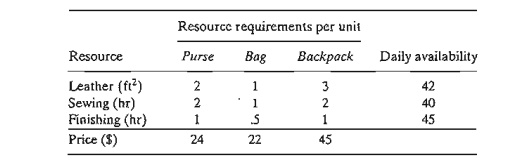Formulate the problem as a linear program and find the optimum solution. Next, indicate whether the following changes in the resources will keep the current solution feasible.

For the cases where feasibility is maintained, determine the new optimum solution (values of the variables and the objective function).

a. Available leather is increased to 45 ft2

b. Available leather is decreased by 1 ft2

c. Available sewing hours are changed to 38 hours.

d. Available sewing hours are changed to 46 hours.

e. Available finishing hours are decreased to 15 hours.

f. Available finishing hours are increased to 50 hours.

g. Would you recommend hiring an additional sewing worker at \$15 an hour?

11. HiDec produces two models of electronic gadgets that use resistors, capacitors, and chips. The following table summarizes the data of the situation:Let x1 and x2 be the amounts produced of Models 1 and 2, respectively. Following are the LP model and its associated optimal simplex tableau.*(a) Determine the status of each resource.

*(b) In terms of the optimal revenue, determine the dual prices for the resistors, capaci-tors, and chips.

(c) Determine the feasibility ranges for the dual prices obtained in (b).

(d) If the available number of resistors is increased to 1300 units, find the new optimum solution.

*(e) If the available number of chips is reduced to 350 units, will you be able to deter-mine the new optimum solution directly from the given information? Explain.

(f) If the availability of capacitors is limited by the feasibility range computed in (c), determine the corresponding range of the optimal revenue and the corresponding ranges for the numbers of units to be produced of Models 1 and 2.

(g) A new contractor is offering to sell HiDec additional resistors at 40 cents each, but only if HiDec would purchase at least 500 units. Should HiDec accept the offer?

12. The 100% feasibility rule. A simplified rule based on the individual changes D1,D2…... , and Dm in the right-hand side of the constraints can be used to test whether or not simultaneous changes will maintain the feasibility of the current solution. Assume that the right-hand side bj of constraint i is changed to bi + D; one at a time, and that PiDi qj is the corresponding feasibility range obtained by using the procedure in Section 3.6.2. By definition, we have Pi ≤; 0 (qj≥ 0) because it represents the maximum allowable decrease (increase) in bi. Next, define r1 to equal D1/P1 if Dj is negative and Di/qi if Di is positive. By definition, we have 0 ≤ r1 ≤ 1. The 100% rule thus says that, given the changesIn reality, the 100% rule is too weak to be consistently useful. Even in the cases where feasibility can be confirmed, we still need to obtain the new solution using the regular simplex feasibility conditions. Besides, the direct calculations associated with simultane-ous changes given in Section 3.6.2 are straightforward and manageable.

To demonstrate the weakness of the rule, apply it to parts (a) and (b) of Problem 1 in this set. The rule fails to confirm the feasibility of the solution in (a) and does not apply in (b) because the changes in Dj arc outside the admissible ranges. Problem 13 further demonstrates this point.

13.     Consider the problemStudy Material, Lecturing Notes, Assignment, Reference, Wiki description explanation, brief detail
Operations Research: An Introduction : The Simplex Method and Sensitivity Analysis : Algebraic Sensitivity Analysis-Changes in the Right-Hand Side |